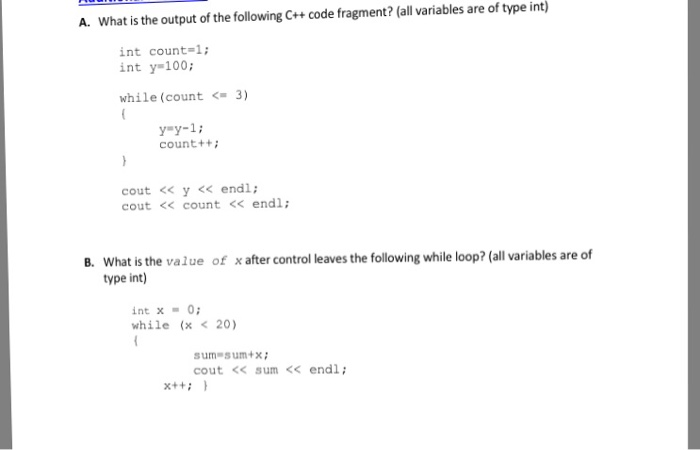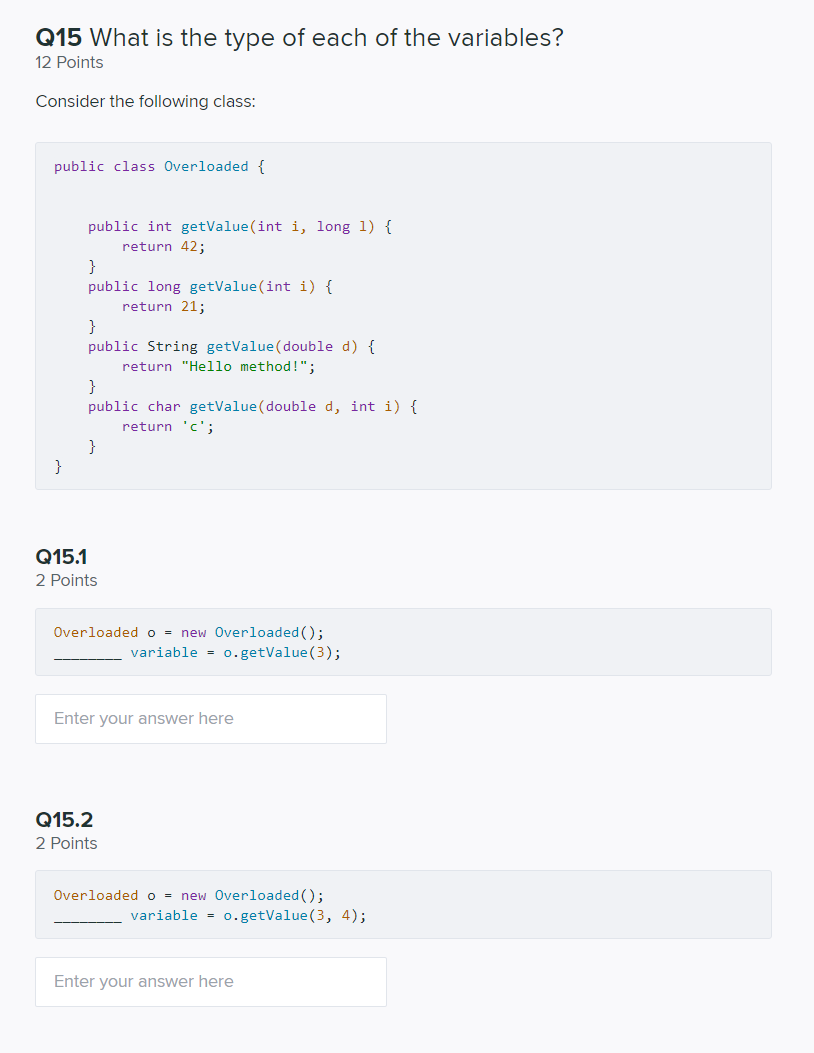# Fix each of the following code by converting the type of the variables: a. double x...

Fix each of the following code by converting the type of the variables: a. double x = 1.5; int y = x b. String x = "1"; Int y = x; c. int x = 1; String y = x; d. double x = 1.0; String y = x;

a. double x = 1.5;

double y = x;

b. String x = "1";

String y = x;

c. int x = 1;

int y = x;

d. double x = 1.0;

double y = x;

#### Earn Coin

Coins can be redeemed for fabulous gifts.

Similar Homework Help Questions
• ### A. What is the output of the following C++ code fragment? (all variables are of type...A. What is the output of the following C++ code fragment? (all variables are of type int) int count-1; int y-100; while (count 3) y=y-1 ; count+t cout << y << endl; cout<< count <<endl What is the value of x after control leaves the following while loop? (all variables are of type int) B. int x0 while (x < 20) sum- sum+x cout << sum<< endl;

• ### What is wrong with this Code? Fix the code errors to run correctly without error. There...

What is wrong with this Code? Fix the code errors to run correctly without error. There are two parts for one code (one question) the two parts branch off, one part has 2 sheets of code, the other has 3 sheets of code, all are small code segments for one question. Pt.1 - 2 sheets of code: public class Computer { private String make; private String type; private String modelNumber; private double cpuSpeed_GHz; private int ramSize_GB; private int hardDriveSize_GB; private...

• ### CONSIDER THE FOLLOWING CODE: double SumString(string x, int n) {       double sum = 0;      ...

CONSIDER THE FOLLOWING CODE: double SumString(string x, int n) {       double sum = 0;       for (int i = 0; i < n; i += 2)             sum += x.length();       return sum; } ///////////////////////////////////////////////////////// void Question() {       string a = "string";       int num = a.length();       double total = SumString(a, num);       cout << "The total is: " << total << endl; } Select one: a. The total is: 14.0 b. The total is: 20.0...

• ### for this code fix the smtax error this is a python code for some reason my...

for this code fix the smtax error this is a python code for some reason my code isnt liking my returns and it bring up other symtax error so fix the following code answer should pop out the following when runned 1/ 2 + 1/ 3 + 1/ 12 = 11/12 z=' ' def math(x,y):     global z     if y==0 or x==0:         return     if y%x ==0:         z=("1/"+str(int(y/x))         return      if x%y ==0         z+=(int(x/y))    ...

• ### Q15 What is the type of each of the variables? 12 Points Consider the following class:...Q15 What is the type of each of the variables? 12 Points Consider the following class: public class Overloaded { public int getValue(int i, long 1) { return 42; } public long getValue(int i) { return 21; } public String getValue(double d) { return "Hello method!"; } public char getValue (double d, int i) { return 'c'; سه مه Q15.1 2 Points Overloaded o = new Overloaded(); variable = 0.getValue(3); Enter your answer here Q15.2 2 Points Overloaded o =...

• ### What is the output of the following code? Is there a relationship between the variables x...

What is the output of the following code? Is there a relationship between the variables x and y? If yes, state the relationship? What is the output? int x = 19683; int i; int y = 0; for (i = x; i >= 1; i = i / 3) y++; cout << "x = " << x << ", y = " << y << endl;

• ### Identify a logical error in the following code and fix it. public class test1 {    ...

Identify a logical error in the following code and fix it. public class test1 {     public static void main(String[] args){         int total;         float avg;         int a, b, c;         a=10;         b=5;         c=2;         total=a+b+c;         avg=total/3;         System.out.println("Average: "+avg);     } } Answer: Write the output of the program below. import java.util.Scanner; public class test2 { public static void main(String[] arg){ int psid; String name; Scanner input=new Scanner(System.in); System.out.println("Enter your PSID"); psid=input.nextInt(); System.out.println("Enter your...

• ### Cant find what is wrong to fix the code. can you please fix the code. The...

Cant find what is wrong to fix the code. can you please fix the code. The function is: sin^-1 (x/5) #include <iostream> #include <iomanip> #include <math.h> #include <cmath> using namespace std; double f(double x) { return pow(sin, -1)*(x / 5); } int main() { int i, n; double a = 0.0, b =1.0, h, s = 0.0, x, xbar; double s1, s2, s3; cout << " Enter # of partitions n "; cin >> n; cout << " n =...

• ### For the following code, which statement is not true? Class Point {     private:         double...

For the following code, which statement is not true? Class Point {     private:         double y;         double z;     public:         double x; }; a.z is available to objects of type Point declared outside the class. b.x is available to objects of type Point declared outside the class. c.x, y, and z are called members variables of the class. d.The name of the class is Point. Given the code below, What is the most accurate description of AwesomeClass(AwesomeClass...